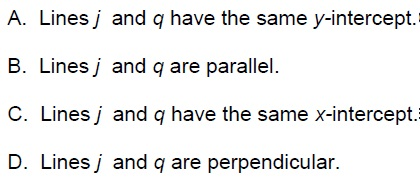ACT enrichment 1
At a local grocery store, watermelons are sold for \$4 each plus an additional \$0.25 per pound. Write a function that describes the relationship between x , the number of pounds of a watermelon, and f (x) , the total cost of the watermelon. *
1 point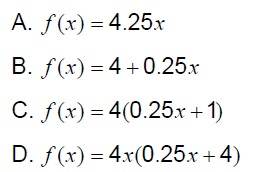What is the value of y in this system *
1 point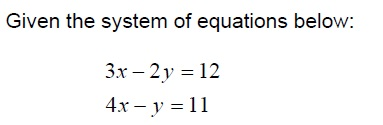Laws of exponents *
1 point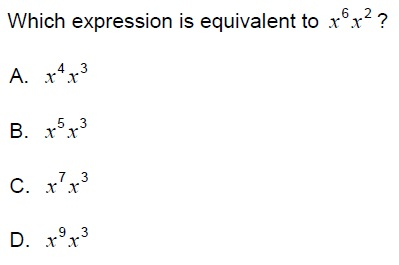linear functions *
1 point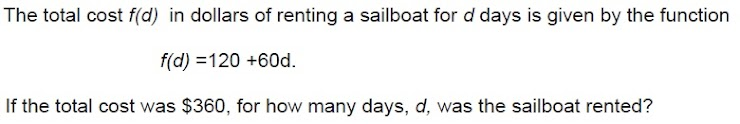Parallel lines(slopes) *
1 point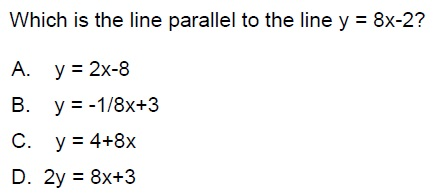Laws of exponents *
1 point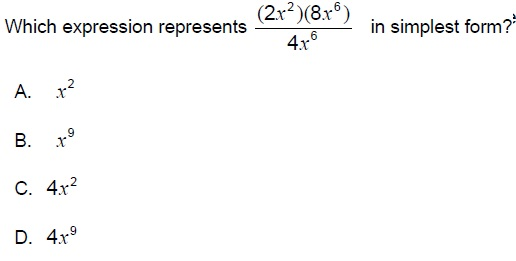Slope *
1 point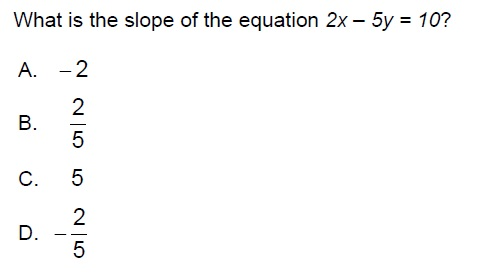The gas tank in Mina’s car holds 15 gallons. Her car gets between 25 and 30 miles to the gallon. If Mina fills up the gas tank and then drives until she runs out of gas, what is the least number of miles she can drive? *
1 point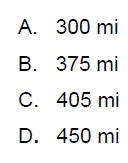polynomial operations *
1 point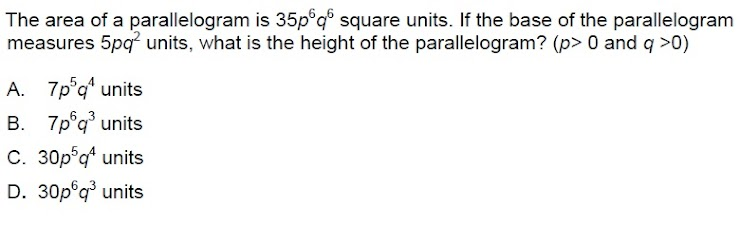The cost of renting a van for one day includes a flat rental fee plus a charge of 45 centsfor each mile the van is driven while it is rented. A van that is driven 107 miles costs \$97.15. A van that is driven 127 miles costs \$106.15. What is the flat rental fee? *
1 point
polynomial operations *
1 point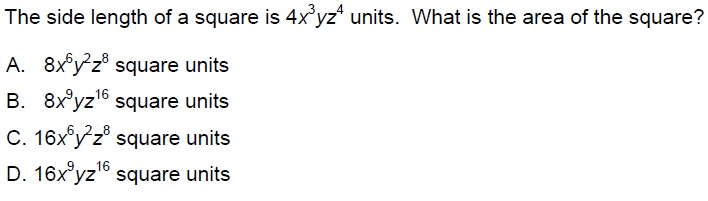period
LAST NAME *
The equation of line j is 6x + 5y = 3, and the equation of line q is 5x – 6y = 0. Which statement about the two lines is true? *
1 point